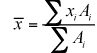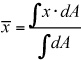##### Statics For DummiesThe centroid or center of area of a geometric region is the geometric center of an object's shape. Centroid calculations are very common in statics, whether you're calculating the location of a distributed load's resultant or determining an object's center of mass. To compute the center of area of a region (or distributed load), you can compute the x-coordinate (and the other coordinates similarly) from the following equations:

• For discrete regions: You can break discrete regions into simple shapes such as triangles, rectangles, circles, and so on.For discrete shapes, creating a simple table such as the one that follows for each coordinate can be useful. You can create as many rows as you need for as many regions as you have.

Sample Table for Calculating Centroids for Discrete Regions
xi Ai xiAi
Region 1 x-distance for Region 1 Area of Region 1 Product of xi and Ai
TOTALS ----------------- Sum of all Ai rows Sum of all xiAi rows
• For continuous regions: Continuous regions are usually defined by more complex boundaries, so you must define them with mathematical equations such as the one that follows: